Include Top

# Case Study 6 – Multiple Response Optimization and Practical Tolerance Design

1. Open the workbook Passive RC Filter Design. DiscoverSim Input Distributions will simulate the variability in control and noise component tolerances. The Normal Distribution (as used in the reference papers) will be specified in cells E17 to E23. The mean values are specified in cells F17 to F23. The standard deviation values are calculated in cells G17 to G23 as discussed above to model the component tolerances, specified in cells H17 to H23. Input Controls will be added to cells F17 to F19 which will vary the mean for the control factors C, R1 and R2 in optimization. The outputs, Deflection and Frequency, will be specified in cells E26 and E27 using the formulas given above.

2.3. Click on cell E17 to specify the Input Distribution for the Capacitor C. Select DiscoverSim > Input Distribution:

4.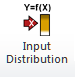5. We will use the default Normal Distribution.

6. Click input Name cell reference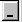and specify cell C17 containing the input name “C”. After specifying a cell reference, the dropdown symbol changes fromto.

7. Click the Mean parameter cell referenceand specify cell F17 containing the mean parameter value = 257.

8. Click the StdDev parameter cell referenceand specify cell G17 containing the standard deviation parameter value = Mean * (Tolerance/100)/3 = (257 * .05)/3 = 4.283.

9. Click Update Chart to view the normal distribution as shown:

10.11. Click OK. Hover the cursor on cell E17 to view the DiscoverSim graphical comment showing the distribution and parameter values:

12.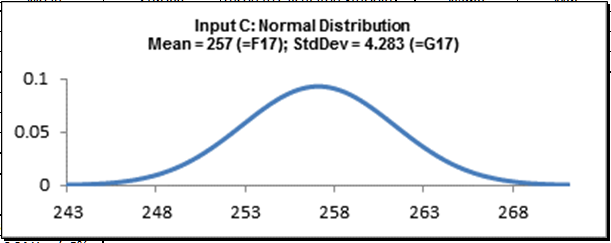13. Click on cell E17. Click the DiscoverSim Copy Cell menu button(Do not use Excel’s Copy – it will not work!).

14. Select cells E18:E23. Click the DiscoverSim Paste Cell menu button(Do not use Excel’s Paste – it will not work!).

15. Review the input comments in cells E18 to E23:

16. Cell E18: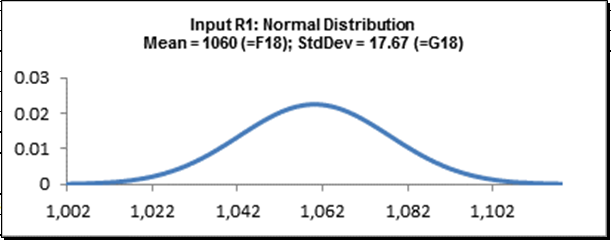Cell E19:Cell E20: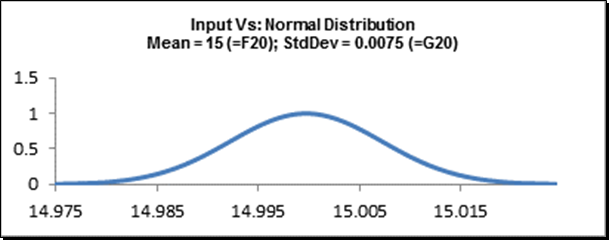Cell E21:Cell E22:Cell E23: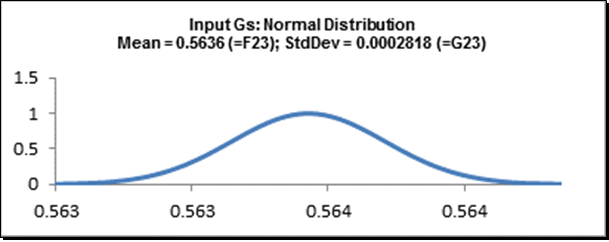17. Now we will specify the outputs. Click on cell E26. Note that the cell contains the Excel formula for Deflection: =(_Vs*_Rg*_R1)/(_Gs*((_R2+_Rg)*(_Rs+_R1)+_Rs*_R1)). Excel range names are used rather than cell addresses to simplify interpretation.

18. Select DiscoverSim > Output Response:

19.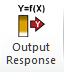20. Enter the output Name as “Deflection”. Enter the Lower Specification Limit (LSL) as 2.97, Target as 3, and Upper Specification Limit (USL) as 3.03. Weight = 1 and Output Goal should be “Target”.

21.Click OK.

22. Hover the cursor on cell E26 to view the DiscoverSim Output information.

23.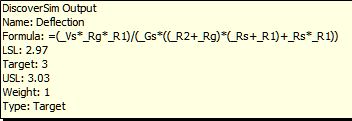24. Click on cell E27. Note that the cell contains the Excel formula for Frequency: =((_R2+_Rg)*(_Rs+_R1)+_Rs*_R1)/(2*PI()*(_R2+_Rg)*_R1*_Rs*_C*0.000001)

25. Select DiscoverSim > Output Response:

26.Click OK

27. Enter the output Name as “Frequency”. Enter the Lower Specification Limit (LSL) as 6.498, Target as 6.84, and Upper Specification Limit (USL) as 7.182. Weight = 1 and Output Goal should be “Target”.Click OK

28. Hover the cursor on cell E27 to view the DiscoverSim Output information.

29.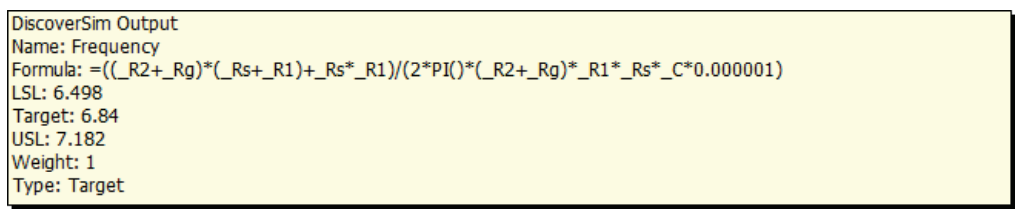30. Now we are ready to perform a simulation run. This is our baseline, after which we will run parameter optimization and compare results. We will also perform a Sensitivity Analysis after optimization.

31. Select DiscoverSim > Run Simulation:

32.33. Select Seed Value and enter “12” as shown, in order to replicate the simulation results given below.

34.Click Run.

35. The DiscoverSim Output Report shows a histogram, descriptive statistics and process capability indices for each output.

36. Deflection Report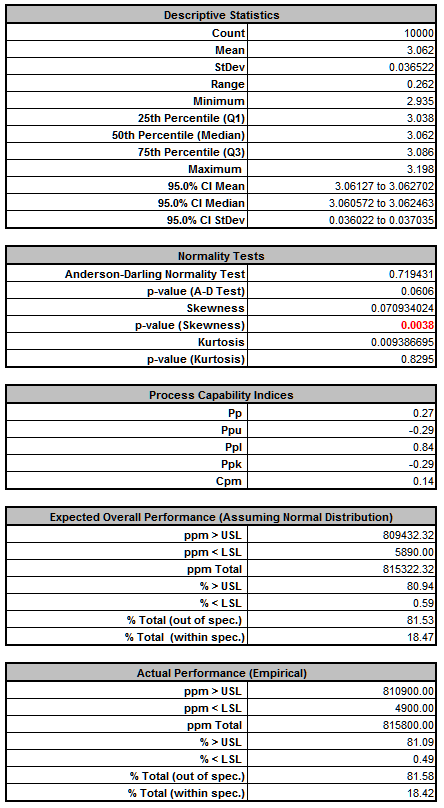From the histogram and capability report we see that the output Deflection is not capable of meeting the specification requirements using the baseline values. The process mean is off target and the variation due to component tolerance is unacceptably large. We expect that 81.5% of the units would fail this requirement, so we must improve the design to center the mean and reduce the variation of Deflection, while simultaneously satisfying the Frequency requirement.

Frequency Report: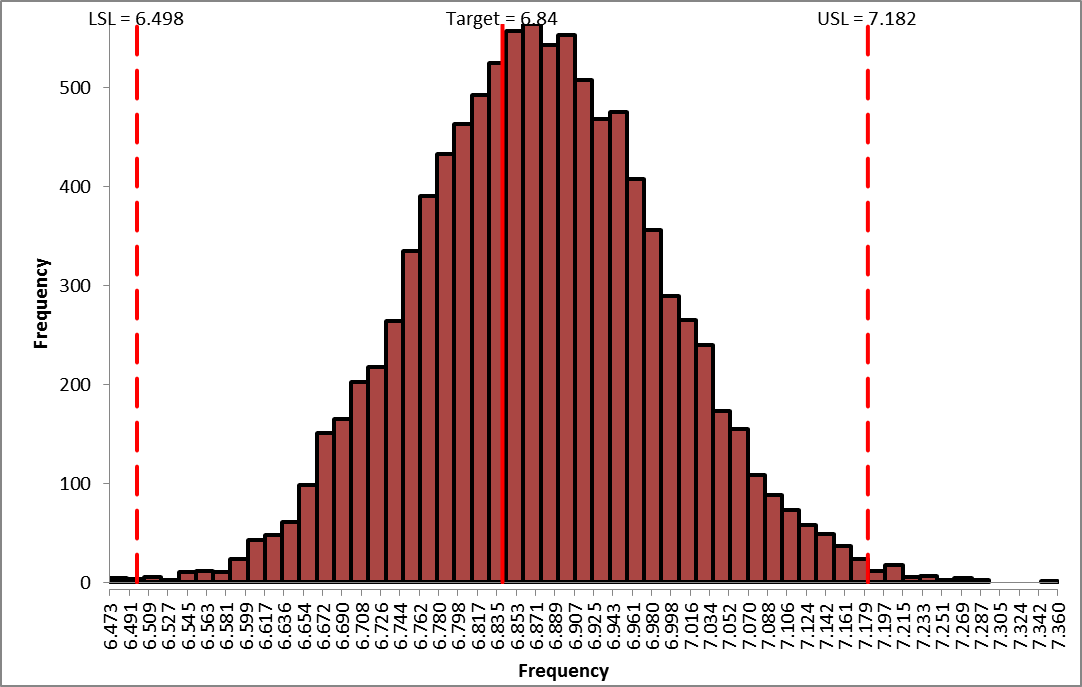From the histogram and capability report we see that the output Frequency is slightly off target and just capable of meeting the specification requirements using the baseline values. We expect that 0.57% of the units would fail this requirement, so if possible we should improve the design to reduce the variation of Frequency while simultaneously improving Deflection.

37. In order to run optimization, we will need to add the Input Controls in cells F17 to F19. Select Sheet1. The Input Control parameters (min/max) are shown below (from Filippone):

38.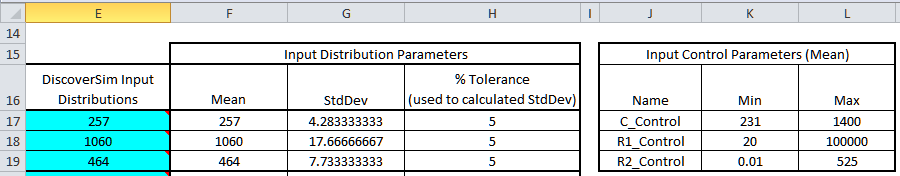These controls will vary the nominal mean values for C, R1 and R2. The standard deviation will be recalculated with each optimization iteration, so that a 5% tolerance is preserved (see above for formula).

39. Click on cell F17. Select Control:40. Click Input Name cell referenceand specify cell J17 containing the input control name “C_Control”.

41. Click the Min value cell referenceand specify cell K17 containing the minimum optimization boundary value = 231.

42. Click the Max value cell referenceand specify cell L17 containing the maximum optimization boundary value = 1400:

43.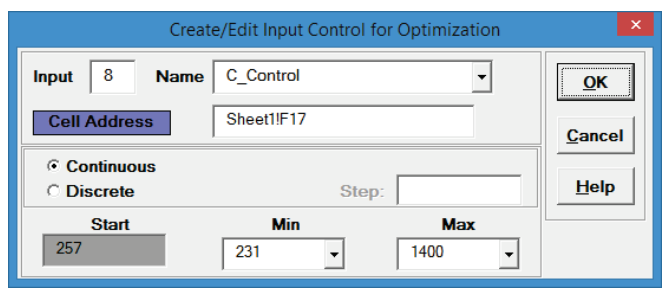44. Click OK. Hover the cursor on cell F17 to view the comment displaying the input control settings:

45.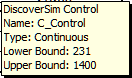46. Click on cell F17. Click the DiscoverSim Copy Cell menu button(Do not use Excel’s Copy – it will not work!).

47. Select cells F18:F19. Click the DiscoverSim Paste Cell menu button(Do not use Excel’s Paste – it will not work!).

48. Review the Input Control comments in cells F17 to F19.

49. The completed model is shown below:

50.51. Now we are ready to perform the optimization. Select DiscoverSim > Run Optimization:

52.53. Select “Minimize” for Optimization Goal, “Weighted Sum” for Multiple Output Metric and “Mean Squared Error” for Statistic. Select Seed Value and enter “12”, in order to replicate the optimization results given below. Set Replications to 1000 to reduce the optimization time. All other settings will be default as shown:

54.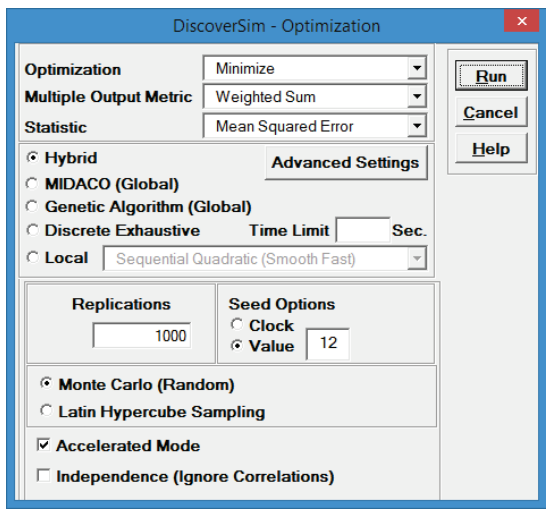Mean Squared Error, also known as a Loss Function, is discussed above:

MSE (Loss) = WeightD[(MeanD – TargetD)2 + StdDevD2] + WeightF[(MeanF – TargetF)2 + StdDevF2]

We want both the Deflection and Frequency outputs to be to be on target with minimal variation. The weights were set when the outputs were created and are the same for each output (= 1).

We will use the Hybrid optimization method which requires more time to compute, but is very powerful to solve complex optimization problems.

55. Click Run. This hybrid optimization will take approximately 2 minutes.

56. The final optimal parameter values are given as:

57.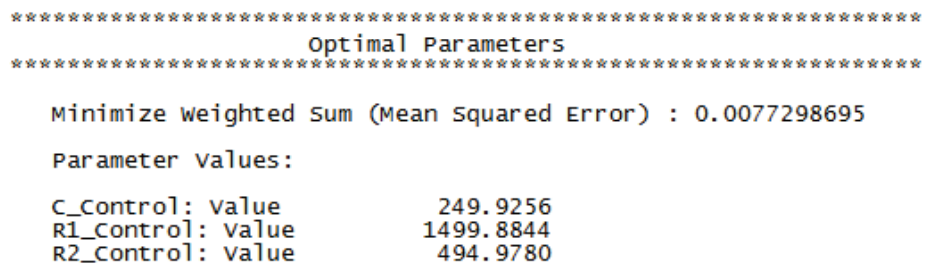58. You are prompted to paste the optimal values into the spreadsheet:

59.Click Yes. This replaces the nominal input control values with the new optimum values. Note that the displayed values for the input distributions at cells E17 to E19 are not overwritten, but when the simulation is run, the referenced mean and standard deviation given in F17 to G19 will be applied.

60. Select Run Simulation. Click Report Options/Sensitivity Analysis. Check Sensitivity Charts and Correlation Coefficients. Select Seed Value and enter “12”, in order to replicate the simulation results given below. Click Run.

61. The DiscoverSim Output Report shows a histogram, descriptive statistics and process capability indices for each output.

62. Deflection Report (after optimization):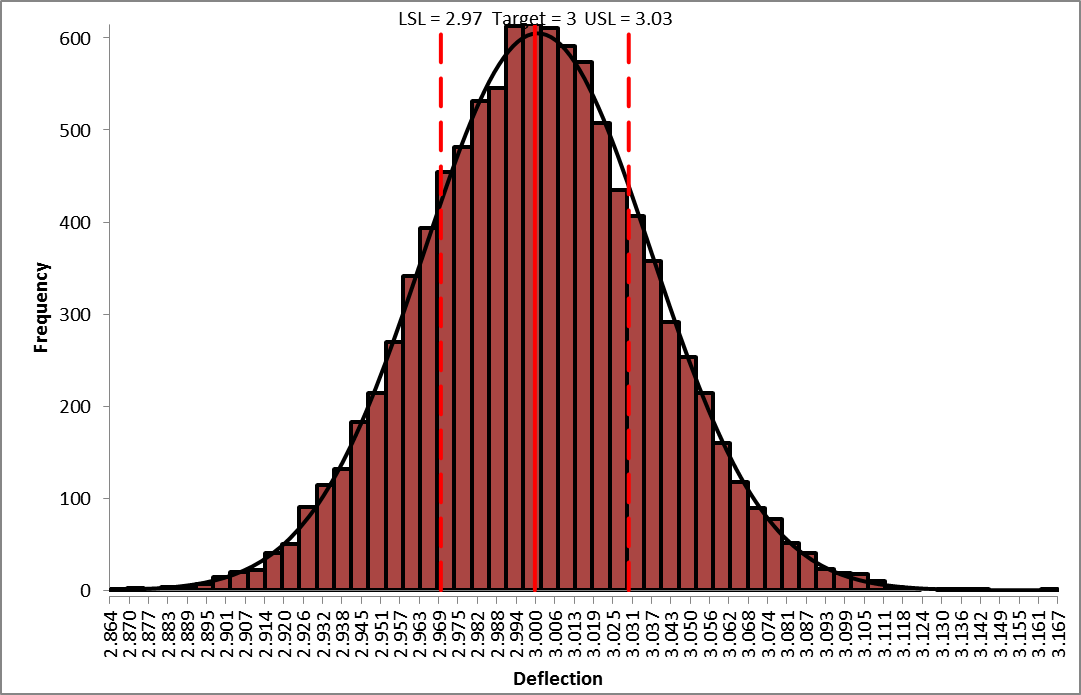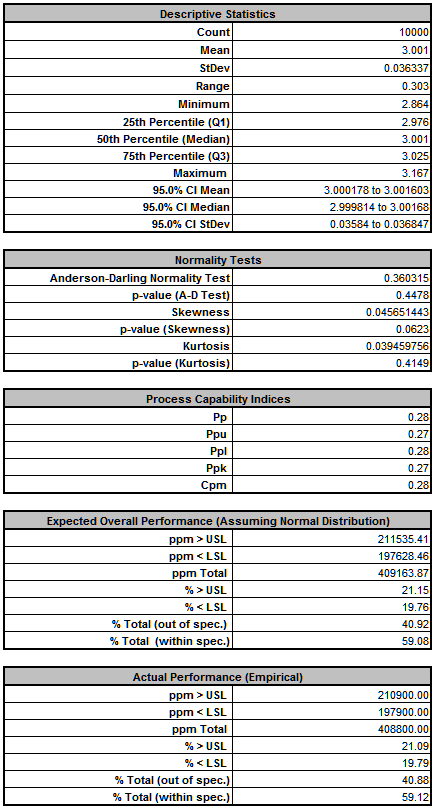From the histogram and capability report we see that the output Deflection is still not capable of meeting the specification requirements after optimization, however the mean is now on target (mean shifted from 6.875 to 6.834). The expected performance failure rate was reduced from 81.5% to 40.9% due to centering the mean. Since we only saw a slight reduction in the standard deviation (0.0365 to 0.0363), the component tolerances will need to be tightened. The sensitivity analysis will help us to focus on which components are critical and require tighter tolerances.

Frequency Report (after optimization):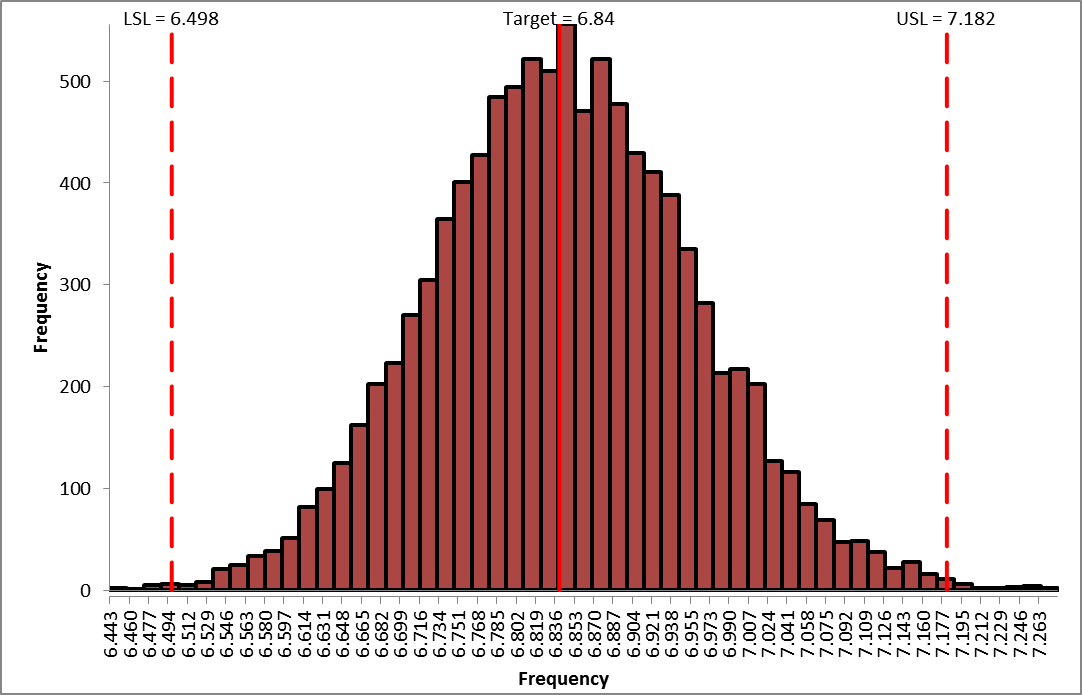From the histogram and capability report we see that the output Frequency after optimization is now on target (mean shifted from 6.875 to 6.844) but still just capable of meeting the specification. The actual performance failure rate was reduced from 0.71% to 0.32% due to centering the mean. Since we did not see a reduction in the standard deviation, the component tolerances will need to be tightened. As with Deflection, the sensitivity analysis will help us to focus on which components are critical and require tighter tolerances.

63. Click on the Sensitivity Correlations sheet:

64.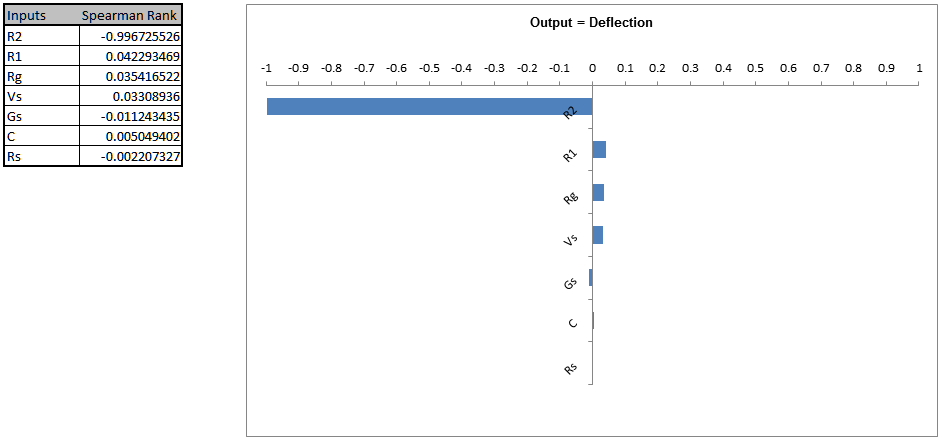Resistor R2 is the dominant component affecting Deflection. A search at the electronic component supplier www.mouser.com, shows that 510 ohm resistors (¼ watt axial) with a 5% tolerance or 1% tolerance, cost approximately \$0.04 each (qty. 100 pricing); resistors with a 0.1% tolerance are \$0.29 to \$1.00 each. Since R2 has such a strong influence on the variability we will use 0.1 % tolerance, even with the higher cost. Afterward, we will consider using 1% tolerance for R2 as a possible cost saving measure. Even though resistor R1 has negligible influence (on Deflection or Frequency below), we will use a 1% tolerance since the price is approximately the same as that of 5% tolerance resistors (5K ohms value).

65. Frequency Sensitivity Chart:

66.Capacitor C is the dominant component affecting Frequency, followed by Resistor R2. Unfortunately, capacitors larger than 230 uF will typically be electrolytic with a 20% tolerance or more. Specifying a tolerance of 5% or less will significantly increase the component cost. Another issue is that the number of available capacitor standard values is very limited compared to that of resistors. We need to reduce the variability of Frequency so we will specify a 2% tolerance (electrolytic > 10V axial). The lowest cost option available to us in the 230 to 300 uF range is a Vishay 280 uF @2%, at \$11.79 each (qty. 101). If this cost is unacceptable then we would need to use a 20% tolerance and relax the output specifications for Frequency or accept the lower process capability/higher defect rate. The cost for a 250uF @20% is \$1.84, qty. 100.

67. Now we will modify the control factor settings and rerun the optimization for practical tolerance design, starting with the fixed capacitor value of 280 uF @2% tolerance. The capacitor is selected first because of the limited selection for available capacitor standard values.

68. Enter the value 280 in control cell F17. Click on cell H17 and change the tolerance value to 2, resulting in a reduced standard deviation value in cell G17 for capacitor C.

69.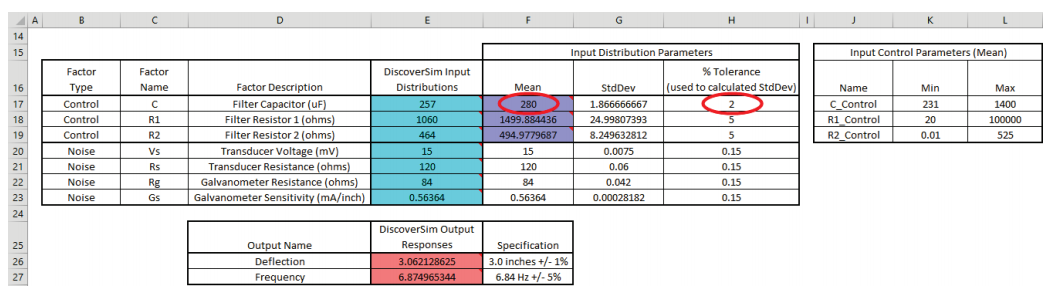Note: The original E17 cell value of 257 for input distribution C is not overwritten in the sheet display, but the referenced control value in F17, 280, will be used in simulation and further optimization.

70. Click on cell H18 and change the tolerance value to 1, resulting in a reduced standard deviation value in cell G18 for resistor R1.

71.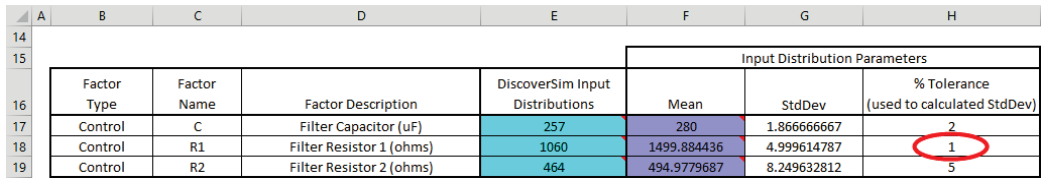72. Click on cell H19 and change the tolerance value to 0.1, resulting in a reduced standard deviation value in cell G19 for resistor R2.

73.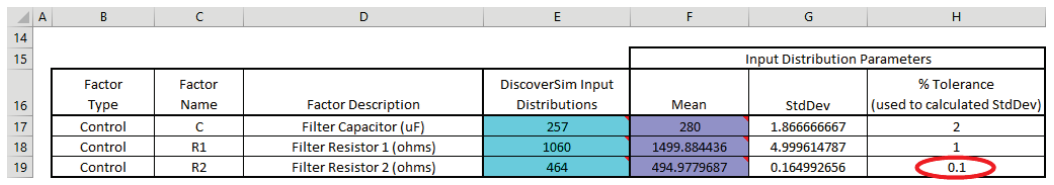74. Enter the Input Control min/max values for C in cells K17 and L17 as 280. This fixes the C value so that it will not vary in optimization.

75.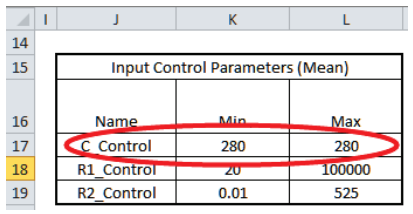76. Now we are ready to perform the optimization. Select DiscoverSim > Run Optimization:

77. Select “Minimize” for Optimization Goal, “Weighted Sum” for Multiple Output Metric and “Mean Squared Error” for Statistic. Select Seed Value and enter “12”, in order to replicate the optimization results given below. Set Replications to 1000 to reduce the optimization time. All other settings will be default as shown:

78.79. Click Run. This hybrid optimization will take approximately 1 minute.

80. The final optimal parameter values are given as:

81.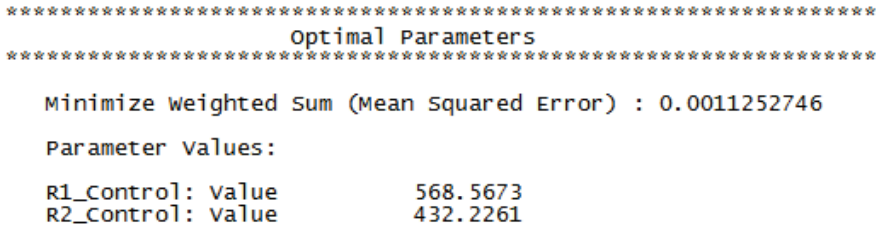82. You are prompted to paste the optimal values into the spreadsheet:

83.Click Yes. This replaces the nominal input control values with the new optimum values.

84. For R2 we will use a ¼ watt axial 432 ohm 0.1% tolerance resistor, available at a cost of 0.64 each (qty 100). Later we will consider using a 432 ohm 1% tolerance resistor as a possible a cost saving measure since they are only .04 each (qty 100).

85. For R1, we will use a ¼ watt axial 565 ohm 1% tolerance resistor for R1, which is the closest available standard value to 566.8 ohms.

86. Enter the value 565 in control cell F18 for R1. The 1.0% tolerance specified in cell H18 does not change. Enter the value 432 in control cell F19 for R2. The 0.1% tolerance specified in cell H19 does not change.

87.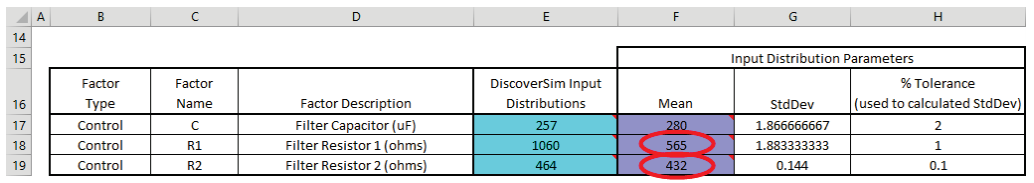88. It is not necessary to “freeze” the R1 and R2 min/max values since we are no longer running optimization.

89. Now type in the C, R1 and R2 values in the Input Distributions cells E17 to E19. Note that this is not required to run the simulation, but allows us to quickly check what the expected values will be for the outputs Deflection (at E26) and Frequency (at E27).

90.91. Now we are ready to perform the simulation. Select Run Simulation. Click Run.

92. The DiscoverSim Output Report shows a histogram, descriptive statistics and process capability indices for each output, and confirms that the RC design has dramatically improved:

93.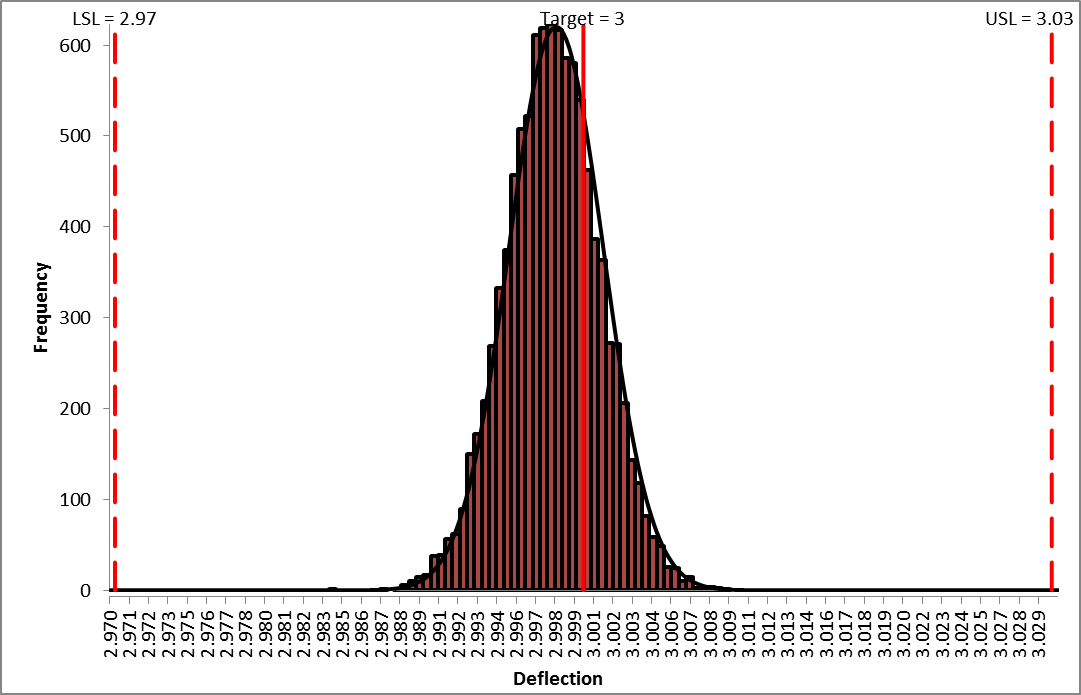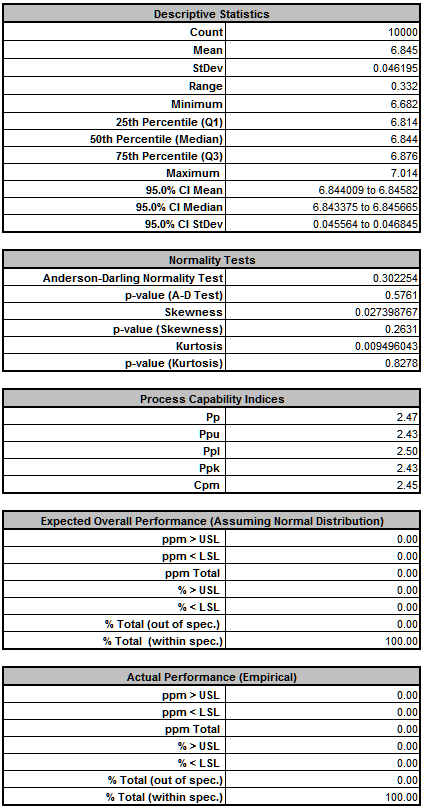94. Now that we have achieved beyond Six Sigma quality for this design (Ppk > 1.5 for each output), we need to consider if we can relax the tolerance for R2 from 0.1% to 1%, thus reducing the component cost (from 0.64 each to .04 each in qty. 100).

95. Click on cell H19 and change the tolerance value from 0.1 to 1, resulting in an increased standard deviation value in cell G19 for resistor R2:

96.97. Now re-run the simulation. Select Run Simulation. Click Run.

98. The resulting simulation report for both outputs is shown below:

99.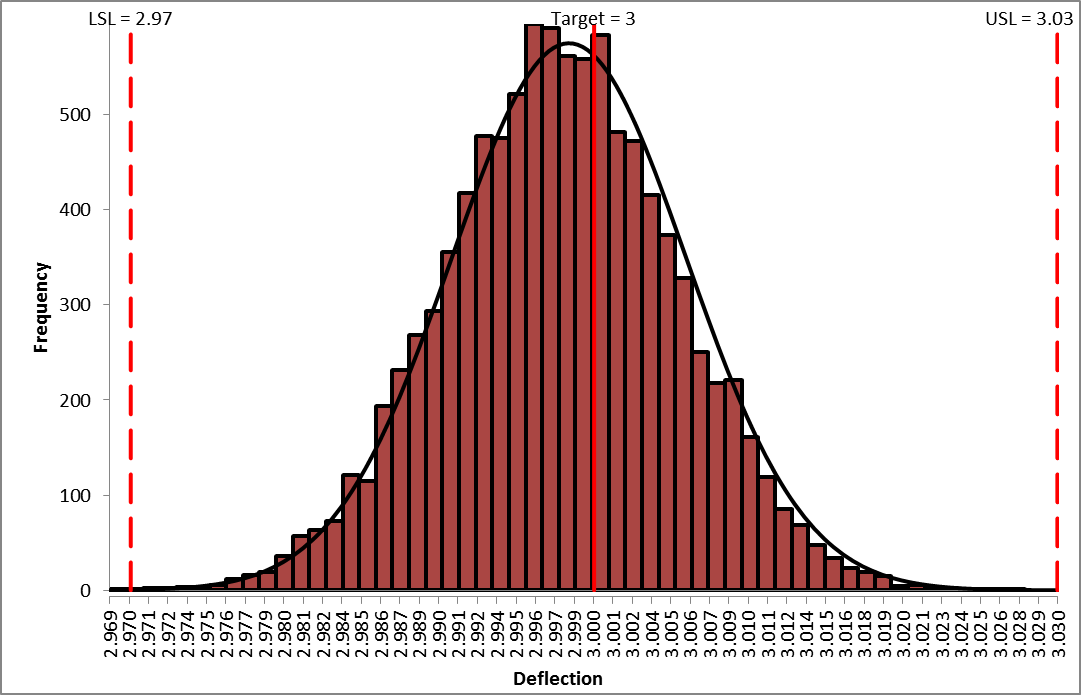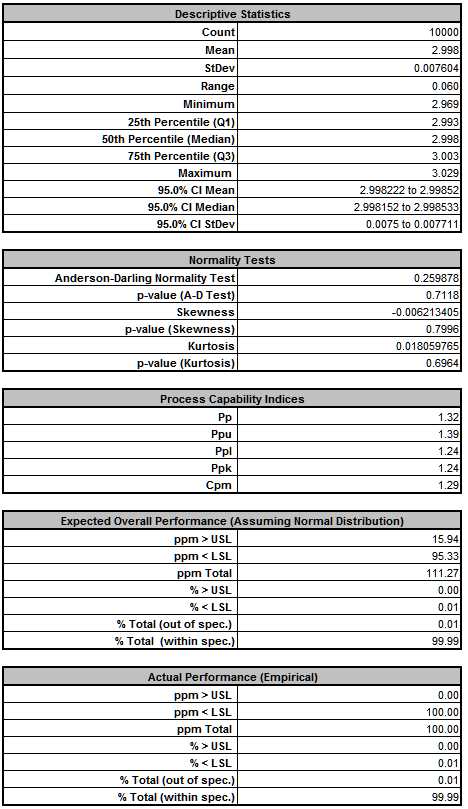As expected from our sensitivity analysis, we see that Ppk for Deflection has dropped from 3.11 to 1.23, and the expected ppm failure rate has increased from 0.0 to 129.84. (Here we also display the Sum of PPM report which includes the sum of ppm values for both Deflection and Frequency).We see that Ppk for Deflection has not changed from 2.43, so increasing the tolerance for R2 from 0.1% to 1% does not have any effect on Deflection.

100. Now that we have run both simulations, we see the trade-off between a difference in predicted ppm failure rate of 129 and a cost difference of 0.60 per unit, thus enabling us to make an informed decision of which tolerance to use for R2.

101. A similar analysis can be performed in the tolerance versus cost consideration for the capacitor.

# Web Demos

Our CTO and Co-Founder, John Noguera, regularly hosts free Web Demos featuring SigmaXL and DiscoverSim